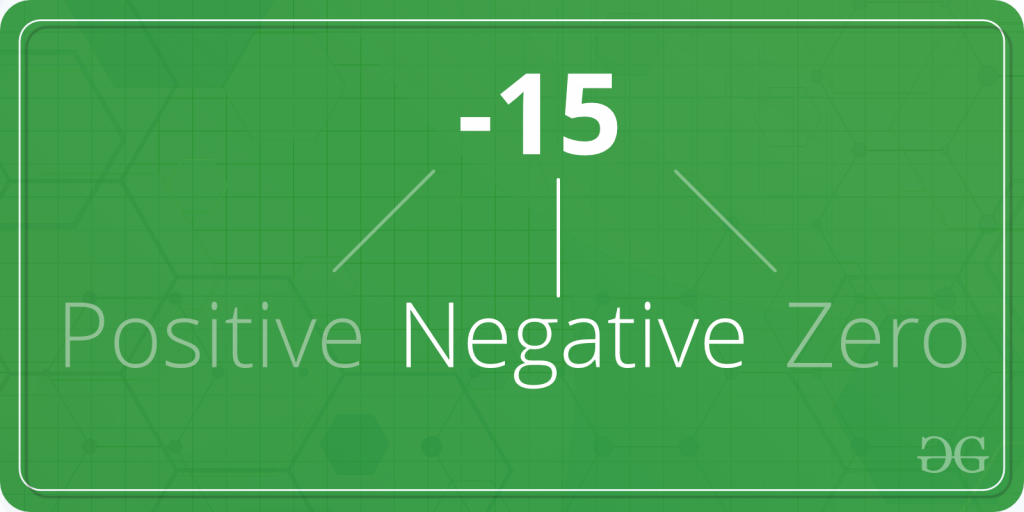# C program to Check Whether a Number is Positive or Negative or Zero

• Difficulty Level : Basic
• Last Updated : 05 Oct, 2018

Given a number A. The task is to check whether A is positive, negative or zero.Take a step-up from those "Hello World" programs. Learn to implement data structures like Heap, Stacks, Linked List and many more! Check out our Data Structures in C course to start learning today.

Examples:

```Input: A = 2
Output: 2 is positive

Input: A = -554
Output: -554 is negative
```

## Recommended: Please try your approach on {IDE} first, before moving on to the solution.

In the below program, to find whether A is positive, negative or zero; first the number is taken as input from the user using scanf in, and then A is checked for positive usingstatement and,andoperators.

Below is the C program to find whether a number is positive, negative or zero.

 `#include `` ` `int` `main()``{``    ``int` `A;`` ` `    ``printf``(``"Enter the number A: "``);``    ``scanf``(``"%d"``, &A);`` ` `    ``if` `(A > 0)``        ``printf``(``"%d is positive."``, A);``    ``else` `if` `(A < 0)``        ``printf``(``"%d is negative."``, A);``    ``else` `if` `(A == 0)``        ``printf``(``"%d is zero."``, A);`` ` `    ``return` `0;``}`

Output:

```Enter the number A =: -54
-54 is negative.
```
My Personal Notes arrow_drop_up